How to Apply the Paired Two-Sample Student's t-Test to Determine if Two Samples have Statistically Different Means

The previous article introduced one-sample Student's t-tests, which involve comparing a set of sample data with a known or predetermined population mean. A similar case involves determining if two correlated samples with the same variance have statistically different means. The approach to this two-sample Student's t-test is similar to the case of one sample, but we add additional assumptions and change the test statistic slightly. This article will review the two-sample Student's t-test and provide an example of the application of this test.

Key Terms

o         Two-sample Student's t-test

Objectives

o         Apply the paired two-sample Student's t-test to determine if two samples have statistically different means

Let's Begin!

In the previous article, we studied one-sample Student's t-tests, which allow us to determine whether the mean of a sample data set deviates in a statistically significant manner from a known or predetermined population mean. In this article, we consider a slightly different case in which we have two samples and wish to determine if they differ in a statistically significant manner: this is the two-sample Student's t-test. (In particular, we will cover paired two-sample t-tests.)

Two-Sample Student's t-Test

Paired two-sample Student's t-tests are useful for cases where each data value in one sample has a corresponding data value in the other sample. A typical example of such data is a study in which samples are collected before and after a certain procedure or event. In such a case, the two-sample t-test can help determine if the procedure or event has any statistically significant effect on the variable by calculating whether a statistically significant variation of the mean has occurred. Paired two-sample t-tests have extensive application in medical science, for example.

The assumptions that underlie two-sample t-tests are similar to those of one-sample t-tests. For example, the variable is assumed to be normally distributed and the data (in a paired sense) chosen at random. In addition, the two sample sets are assumed to be correlated, and they are also assumed to have the same sample variance.

The null hypothesis for the two-sample t-test is slightly different from the one-sample version, but the basic idea is the same. The null and corresponding alternative hypotheses are (some form of) the following:

H0 = The sample means do not differ significantly.

Ha = The sample means differ significantly.

The critical value (c) for a given significance level (α, which, again, is usually 0.05 or 0.01) is determined using the same table of values, and the number of degrees of freedom is once again the sample size minus one.

The test statistic for the two-sample Student's t-test is slightly different, but it follows the same general idea. If we assign the variable name X to one sample set and Y to the other, then the value of t (the test statistic) is the following, where n is the sample size and s is the sample standard deviation (recall that we assume that the two samples have the same variance).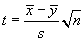An alternative formula uses the variable D, which is defined as X – Y. The test statistic in this case relies on the mean of D (which is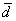) and the sample standard deviation of D (which is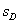).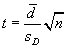Once again, if the value of t exceeds the critical value c, then we reject the null hypothesis and proceed on the assumption that the alternative hypothesis is correct. Otherwise, we do not reject the null hypothesis. The following example illustrates how the two-sample t-test can be used.

A certain medical experiment involves giving a group of randomly selected patients a drug to determine if that drug has a significant effect on a certain blood measurement. Let's call this measurement before the drug is administered X, and we'll call it Y for the data collected after it is administered. Note that unlike the one-sample t-test, we do not need a population mean-we are simply comparing two sample means.

Our null hypothesis in this case is the same as above: the sample means do not differ significantly. We'll choose a significance level of 0.01 for this case. The hypothetical data for two sets of measurements are given in the table below.

Interested in learning more? Why not take an online Applied Statistics course?

 X Y 1.02 1.04 1.05 1.04 1.09 1.10 0.98 0.97 0.97 1.01 1.01 1.07

Now, let's calculate the sample means.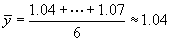The variance of X is the following. Although we can show that the variances of the samples are very nearly the same, we will simply proceed on the assumption that they are the same.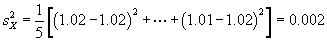The standard deviation is then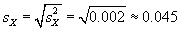We can now calculate the test statistic for the data as follows.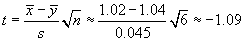Since the negative sign is not meaningful in this context, we ignore it and use t = 1.09. We can now look up the critical value, c, for the number of degrees of freedom (five) in the problem and the statistical significance level (0.01). We can use the same Student's't table of values; the result is given below.

c = 3.36

Since t does not exceed c, we are justified in not rejecting our null hypothesis: the two sample means do not differ significantly. Thus, were this a medical trial, the scientist might conclude that the drug does not have any statistically significant effect on the blood parameter measured in the experiment.

Practice Problem: Determine if the two samples in the data set below have a means that differ significantly to a statistical significance level of 0.05.

 X Y 25 26 26 27 24 25 29 30 27 28 26 27 23 24 28 29

Solution: First, let's calculate the sample mean in each case. Our null hypothesis is that these means do not differ in a statistically significant manner.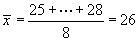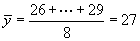The sample variance for X is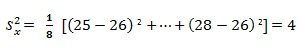The sample standard deviation is then the following. We will use this value when calculating the test statistic.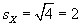The test statistic for the two-sample Student's t-test is the following.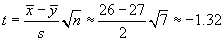Ignoring the negative sign, we get a value of t = 1.32. We must now use the table of Student's't values to find the critical value for seven degrees of freedom and a significance level of 0.05. The result is given below.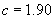Once again, the test statistic does not exceed the critical value, so we do not reject the null hypothesis in this case.